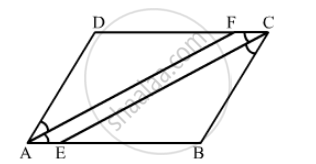Advertisement Remove all ads

# In Fig. 17.31, Abcd is a Parallelogram, Ce Bisects ∠C and Af Bisects ∠A. in Each of the Following, If the Statement is True, Give a Reason for the Same: - Mathematics

Sum

In the following  Figure  ABCD is a  arallelogram, CE bisects ∠C and AF bisects ∠A. In each of the following, if the statement is true, give a reason for the same:(i) ∠A = ∠C
(ii) $\angle FAB = \frac{1}{2}\angle A$

(iii) $\angle DCE = \frac{1}{2}\angle C$

(iv) $\angle CEB = \angle FAB$

(v) CE || AF

Advertisement Remove all ads

#### Solution

(i) True, since opposite angles of a parallelogram are equal.
(ii) True, as AF is the bisector of$\angle$ A.

(iii) True, as CE is the bisector of $\angle$C.

(iv) True

$\angle$CEB =$\angle$ DCE........(i)  (alternate angles)

$\angle$DCE= $\angle$ FAB.........(ii) (opposite angles of a parallelogram are equal)

From equations (i) and (ii):

$\angle$ CEB =$\angle$FAB

(v) True, as corresponding angles are equal ($\angle$ CEB =$\angle$ FAB).

Is there an error in this question or solution?
Advertisement Remove all ads

#### APPEARS IN

RD Sharma Class 8 Maths
Chapter 17 Understanding Shapes-III (Special Types of Quadrilaterals)
Exercise 17.1 | Q 26 | Page 12
Advertisement Remove all ads

#### Video TutorialsVIEW ALL 

Advertisement Remove all ads
Share
Notifications

View all notifications

Forgot password?
Course